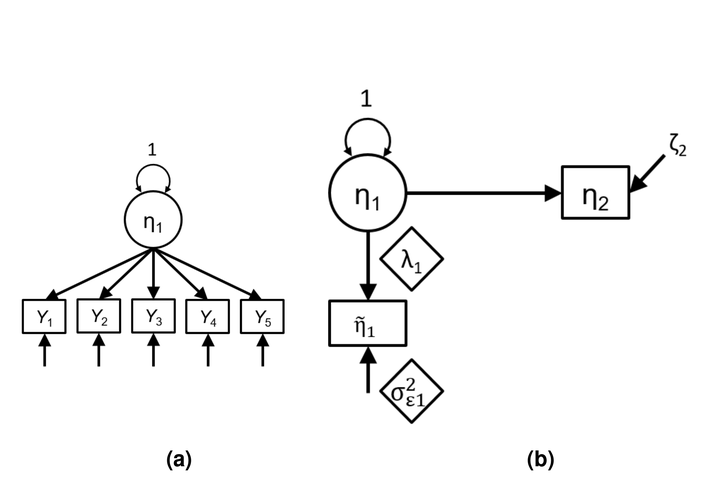# Two-stage path analysis with definition variables: An alternative framework to account for measurement error### Abstract

When estimating path coefficients among psychological constructs measured with error, structural equation modeling (SEM), which simultaneously estimates the measurement and structural parameters, is generally regarded as the gold standard. In practice, however, researchers usually first compute composite scores or factor scores, and use those as observed variables in a path analysis, for purposes of simplifying the model or avoiding model convergence issues. Whereas recent approaches, such as reliability adjustment methods and factor score regression, has been proposed to mitigate the bias induced by ignoring measurement error in composite/factor scores with continuous indicators, those approaches are not yet applicable to models with categorical indicators. In this paper, we introduce the two-stage path analysis (2S-PA) with definition variables as a general framework for path modeling to handle categorical indicators, in which estimation of factor scores and path coefficients are separated. It thus allows for different estimation methods in the measurement and the structural path models and easier diagnoses of violations of model assumptions. We conducted three simulation studies, ranging from latent regression to mediation analysis with categorical indicators, and showed that 2S-PA generally produced similar estimates to those using SEM in large samples, but gave better convergence rates, less standard error bias, and better control of Type I error rates in small samples. We illustrate 2S-PA using data from a national data set, and show how researchers can implement it in Mplus and OpenMx. Possible extensions and future directions of 2S-PA are discussed.

Type
Publication
Psychological Methods. Advance online publication